Custom SearchSUMS The integral of an algebraic sum of differentiable functions is the same as the algebraic sum of the integrals of these functions taken separately; that is, the integral of a sum is the sum of the integrals. Formula.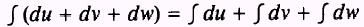PROOF:Therefore,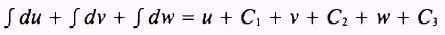whereThenand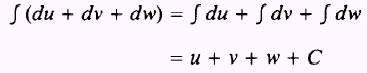EXAMPLE: Evaluate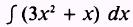SOLUTION:EXAMPLE: Evaluate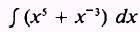SOLUTION: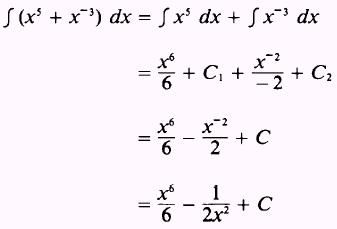PRACTICE PROBLEMS: Evaluate the following integrals:ANSWERS: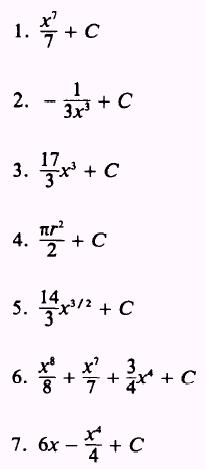Integrated Publishing, Inc. - A (SDVOSB) Service Disabled Veteran Owned Small Business